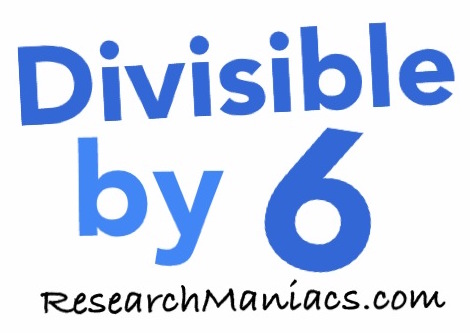Divisible by 6Welcome to Research Maniacs' "Divisible by 6" lookup page. When you enter a number on this lookup page, we will do the following.

1 - We will of course give you the yes/no answer to whether your number is divisible by 6 or not.

2 - We will tell you what your number divided by 6 actually is (the quotient).

3 - AND most importantly, we will show you how we determined the answer so you can do it yourself in the future.

To get started, simply enter your number in the box below:

Not sure what number to check, huh? If you are not sure what to enter above, try one of these:

Is 26 divisible by 6?

Is 66 divisible by 6?

Is 99 divisible by 6?

Is 247 divisible by 6?